# How does a pressure transmitter work?

To understand how a pressure transmitter works, it is enough to focus on its two key elements: One is the pressure sensor, which detects the pressure of the medium and converts it into an electrical signal. The other is an application specific integrated microchip. It is needed to convert the generated electrical signal into a normalised output signal.

## Pressure sensor

The pressure sensor consists of a thin-film-on-steel cell (Figure 1), where the resistance bridges are applied as a thin film to the surface of a steel sensing element. This thin film is only a few atomic layers thick. When pressure is applied to the pressure sensor, its membrane deforms at predefined points. The resistors are placed exactly at these points and change their value when stretched or compressed. There are four strain resistors on the sensing element. Two resistors each form a path. A bridge can be formed in the middle where the voltage can be measured. This bridge is called a Wheatstone bridge. When there is no pressure, all the resistors have the same value, so there is no voltage between the left and right paths.

When pressure deforms the membrane, two resistors are compressed, and two resistors are stretched. This increases the electrical resistance in the stretched areas (Figures 2 and 3). On the other hand, the pressure decreases in the compressed areas. This changes the state of the resistance bridge, and a signal is generated. However, the measured signal is not linear and varies depending on the ambient temperature. This is because the temperature has a strong influence on the resistance of the bridge (Figure 4).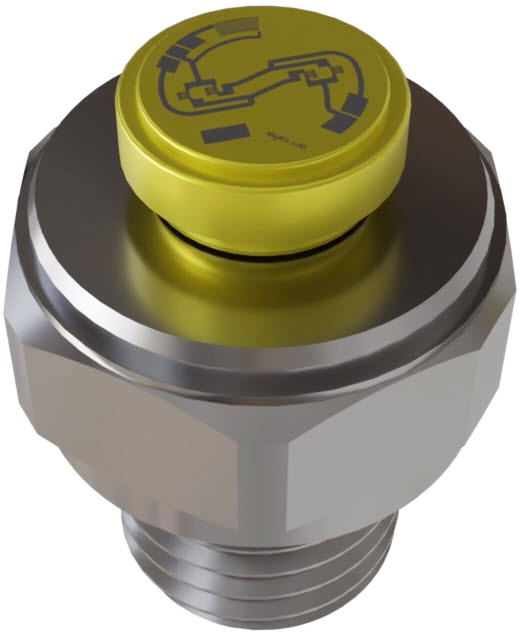Figure 1: Pressure sensor, a thin-film on steel sensing element with resistance bridges.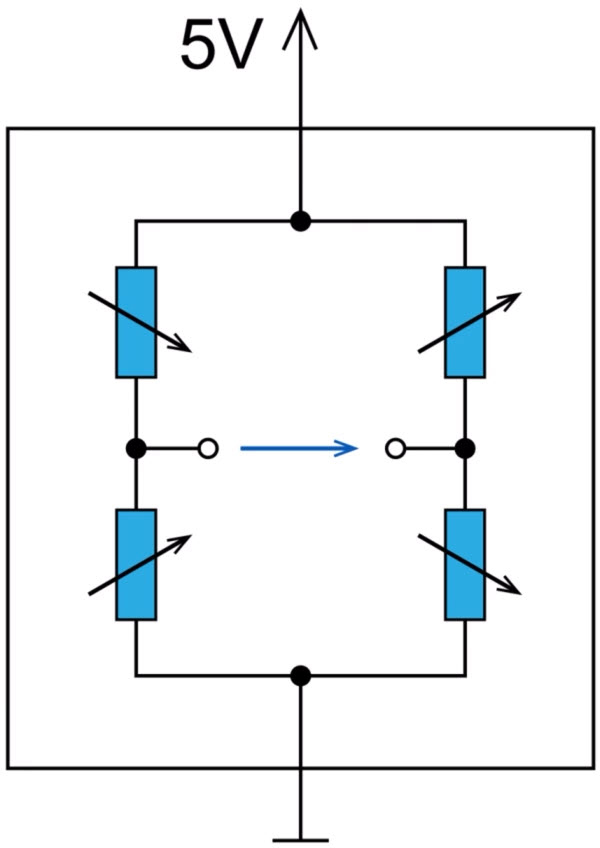Figure 2: Schematic diagram of the Wheatstone bridge with its four resistors (left).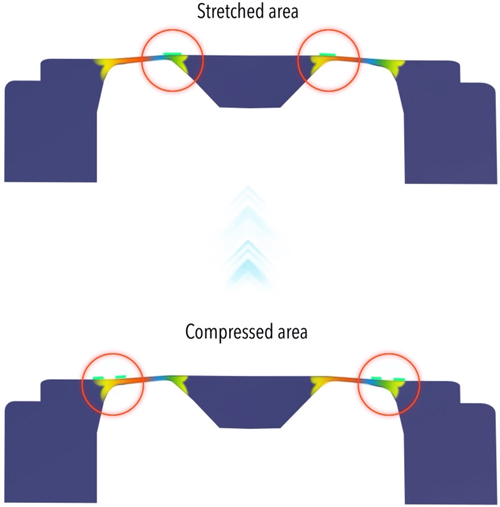Figure 3: When pressure is applied, two resistors are stretched (top) and two are compressed (bottom).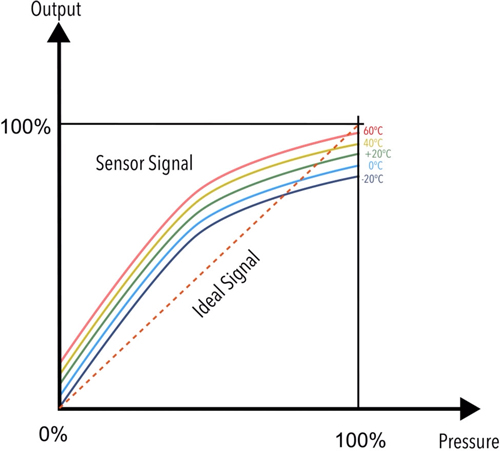Figure 4: The measured signal varies with temperature. Intelligent electronics are needed to correct this.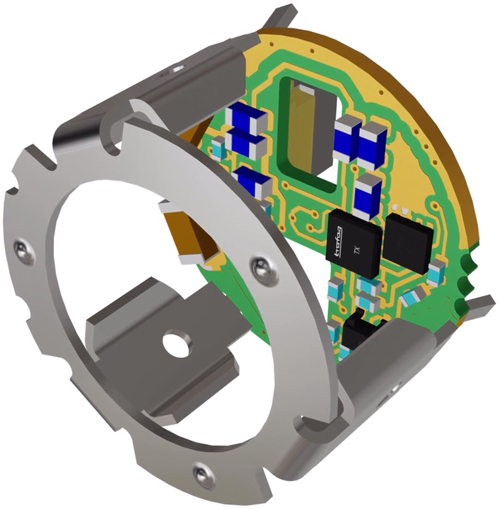Figure 5: The Application Specific Integrated Circuit (ASIC) is connected to the PCB via solder points (black cube marked "trafag" and "TX"). It corrects and amplifies the measured signals.

## Application specific microchip

To obtain a linear, accurate and temperature independent measurement signal from the measured signal, intelligent electronics are required. The electronics corrects and amplifies the measurement signal, e.g., a 10-millivolt signal is transformed into a 10-Volt signal. The correction values obtained are stored in the application specific microchip (also called Application Specific Integrated Circuit - ASIC, Figure 5). These values are determined and stored individually for each pressure transmitter. To determine the correction values, a precisely defined pressure is applied to the ready-mounted pressure transmitter and the signal is measured. For the applied pressure, the correction values can be calculated. The process is then repeated at different temperatures. This allows the correction values for temperature compensation to be determined. The correction values determined in this way are then stored in the chip. In this way, a linear and standardised measurement signal can be generated from the raw signal of the sensing element. And this over the entire pressure and temperature range (Figure 6). This standardised measurement signal can be transmitted to higher-level control systems.

The application-specific microchip (ASIC), houses millions of circuits on an area of approximately 2.5 x 2.5 millimeters, where soldering points establish contact between the chip and the electronics of the pressure transmitter (Figure 7).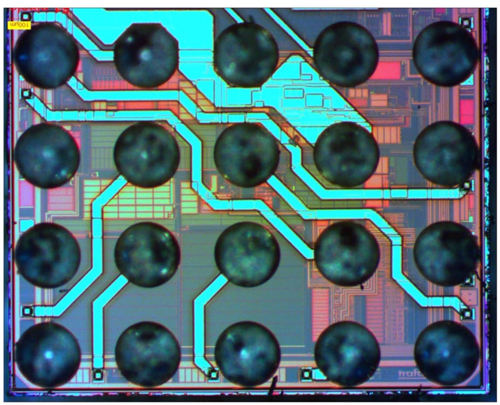Figure 7: The ASIC, contains millions of circuits on an area of about 2.5 x 2.5 millimeters. The solder points (grey-blue circles in the picture) provide the contact between the chip and the electronics of the pressure transmitter.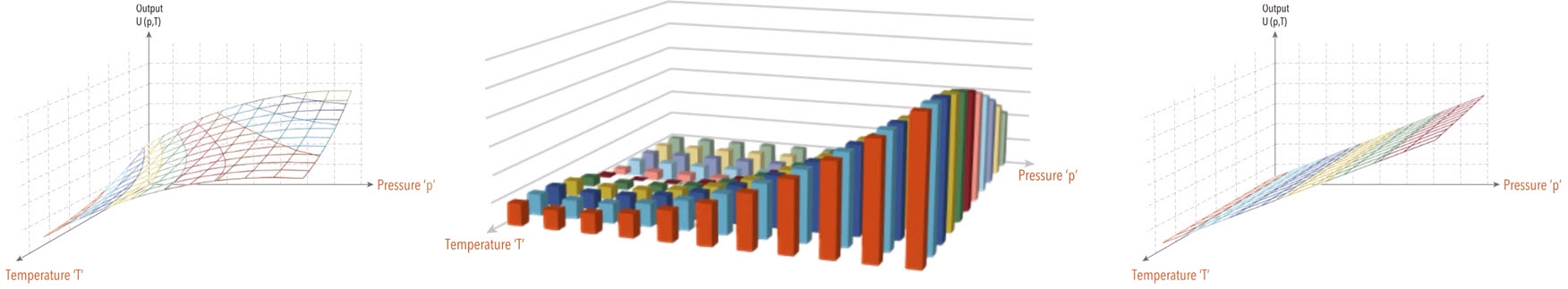Figure 6: The raw signal (left) and the correction values (middle) are added to obtain the standardised output signal (right).

## Conclusion

The best measuring results are obtained when the measuring cell and the microchip are precisely matched. For this reason, Trafag produces its own measuring cells and has developed its own ASIC. By developing these two key components under one roof, the pressure transmitter functions optimally, and quality and reliability can be guaranteed.

Do you have any questions about the design of the Trafag pressure transmitter?